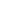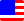Documents  >  Class  >  real
real class

Description

Represents a 64 bit floating point number.

Notes

This class is a standard built-in class in the Rice language.

Instance that is created by declaration have been already initialized.

The initial value is 0.0.

The maximum value is 1.79769313486232 e 308.

The minimum value is -1.79769313486232 e 308.

fitter

 Signature Description real() None. real(int) Get a new instance of real class with the same value as the argument. real(long) Get a new instance of real class with the same value as the argument. real(real) Get a new instance of real class with the same value as the argument.

getter

 Signature Description B Get an instance of bool type with converted value from real value. Epsilon Represents the smallest positive Double value that is greater than zero. Fitted Gets the value that indicates whether this instance was already initialized. I Gets an instance of int type that have a value that truncate the fractional part. L Gets an instance of long type that have a value that truncate the fractional part. Max Returns the maximum value of the real type. Min Returns the minimum value of the real type. NaN Represents the value that is not a number (NaN). NegativeInfinity Represents negative infinity. PositiveInfinity Represents positive infinity. R Gets an another instance of the real type with the same value. S Gets a string representation of the real value. TypeName Gets the type name.

setter

 Signature Description Value(real) This setter will change only the value without changing the reference value.

method

 Signature Description Ceiling() Gets an new instance of the real type that has the smallest integral value that is greater than or equal to the instance value. Clone() Gets a new instance with the same value as the calling instance. Floor() Gets an new instance of the real type that has the largest integer less than or equal to the instance value. IsInfinity() Gets a value that indicates whether this instance value evaluates to negative or positive infinity. IsNaN() Gets a value that indicates whether this instance value is not a number (NaN). IsNegativeInfinity() Gets a value that indicates whether this instance value evaluates to negative-infinity. IsPositiveInfinity() Gets a value that indicates whether this instance value evaluates to positive-infinity. Round() Gets an new instance of the real type that has the nearest integral value to the instance value. Round2() Gets an new instance of the real type that has the nearest integral value to the instance value. ToString() Gets a string representation of the real value. Truncate() Gets an new instance of the real type that has a nearest integer value towards zero from the instance value.
Copyright © Rice All rights reserved.